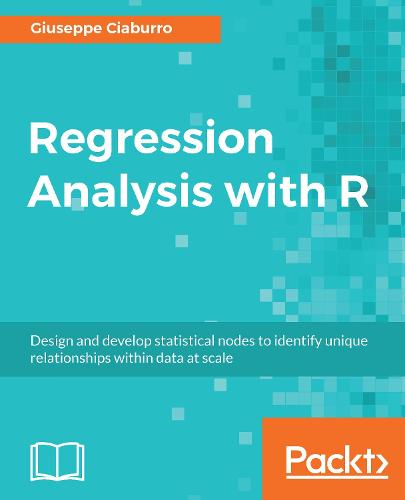•# Regression Analysis with R: Design and develop statistical nodes to identify unique relationships within data at scale (Paperback)

(author)
£29.99
Paperback 422 Pages / Published: 31/01/2018
• We can order this

Usually dispatched within 10 working days

Build effective regression models in R to extract valuable insights from real data

Key FeaturesImplement different regression analysis techniques to solve common problems in data science - from data exploration to dealing with missing valuesFrom Simple Linear Regression to Logistic Regression - this book covers all regression techniques and their implementation in RA complete guide to building effective regression models in R and interpreting results from them to make valuable predictionsBook Description

Regression analysis is a statistical process which enables prediction of relationships between variables. The predictions are based on the casual effect of one variable upon another. Regression techniques for modeling and analyzing are employed on large set of data in order to reveal hidden relationship among the variables.

This book will give you a rundown explaining what regression analysis is, explaining you the process from scratch. The first few chapters give an understanding of what the different types of learning are - supervised and unsupervised, how these learnings differ from each other. We then move to covering the supervised learning in details covering the various aspects of regression analysis. The outline of chapters are arranged in a way that gives a feel of all the steps covered in a data science process - loading the training dataset, handling missing values, EDA on the dataset, transformations and feature engineering, model building, assessing the model fitting and performance, and finally making predictions on unseen datasets. Each chapter starts with explaining the theoretical concepts and once the reader gets comfortable with the theory, we move to the practical examples to support the understanding. The practical examples are illustrated using R code including the different packages in R such as R Stats, Caret and so on. Each chapter is a mix of theory and practical examples.

By the end of this book you will know all the concepts and pain-points related to regression analysis, and you will be able to implement your learning in your projects.

What you will learnGet started with the journey of data science using Simple linear regression Deal with interaction, collinearity and other problems using multiple linear regressionUnderstand diagnostics and what to do if the assumptions fail with proper analysisLoad your dataset, treat missing values, and plot relationships with exploratory data analysis Develop a perfect model keeping overfitting, under-fitting, and cross-validation into considerationDeal with classification problems by applying Logistic regressionExplore other regression techniques - Decision trees, Bagging, and Boosting techniquesLearn by getting it all in action with the help of a real world case study.Who this book is for

This book is intended for budding data scientists and data analysts who want to implement regression analysis techniques using R. If you are interested in statistics, data science, machine learning and wants to get an easy introduction to the topic, then this book is what you need! Basic understanding of statistics and math will help you to get the most out of the book. Some programming experience with R will also be helpful

Publisher: Packt Publishing Limited
ISBN: 9781788627306
Number of pages: 422
Dimensions: 93 x 75 mm